# High School Chemistry : Identifying Unknown Concentration

## Example Questions

2 Next →

### Example Question #471 : High School Chemistry

30mL of a solution of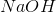of unknown concentration mixed with 19mL of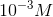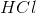produced a solution with a pH of 7. What is the concentration of thesolution?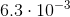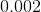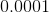None of these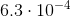Explanation:

For this question use the following formula: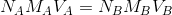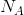is the number of acidic hydrogens on the acid,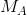is the molarity of the acid,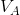is the volume of the acid,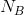is the number of basic hydroxides on the base,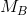is the molarity of the base,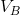is the volume of the base

Rearrange the equation for the molarity of the base: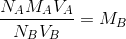Plug in known values and solve.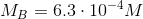### Example Question #481 : High School Chemistry

29mL of a solution of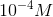is mixed with 65mL of ansolution of unknown concentration produced a solution with a pH of 7. What is the concentration of thesolution?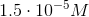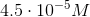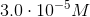None of theseExplanation:

For this question use the following formula:is the number of acidic hydrogens on the acid,is the molarity of the acid,is the volume of the acid,is the number of basic hydroxides on the base,is the molarity of the base,is the volume of the base

Rearrange the equation for the molarity of the acid: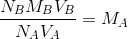Plug in known values and solve.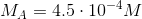### Example Question #482 : High School Chemistry

39mL of a solution ofis mixed with 24mL of ansolution of unknown concentration produced a solution with a pH of 7. What is the concentration of thesolution?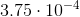None of these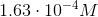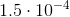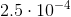Explanation:

For this question use the following formula:is the number of acidic hydrogens on the acid,is the molarity of the acid,is the volume of the acid,is the number of basic hydroxides on the base,is the molarity of the base,is the volume of the base

Rearrange the equation for the molarity of the acid:Plug in known values and solve.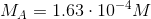### Example Question #71 : Acid Base Chemistry

It takes 25mL of a .001Mto neutralize 5mL of a solution ofof unknown concentration. What is the concentration of the unknown solution?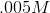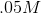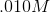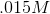None of theseExplanation:

For this question use the following formula:is the number of acidic hydrogens on the acid,is the molarity of the acid,is the volume of the acid,is the number of basic hydroxides on the base,is the molarity of the base,is the volume of the base

Rearrange the equation for the molarity of the acid:Plug in known values and solve.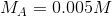### Example Question #484 : High School Chemistry

It takes 35mL of .01Mto neutralize 5mL of a solution ofof unknown concentration. What is the concentration of the unknown solution?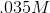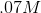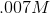None of these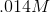Explanation:

For this question use the following formula:

is the number of acidic hydrogens on the acid,  is the molarity of the acid,  is the volume of the acid,  is the number of basic hydroxides on the base,  is the molarity of the base,  is the volume of the base

Rearrange the equation for the molarity of the acid:

Plug in known values and solve.

2 Next →

### All High School Chemistry Resources